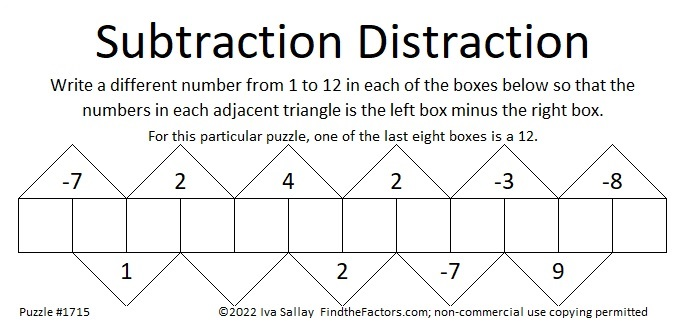# 1715 A Lot More of a Subtraction Distraction

Contents

### Today’s Puzzle:

Last time I published a puzzle with the last clue missing. Leaving out the first or the last clue only makes the puzzle slightly more difficult. What if I left out a clue more in the middle of the puzzle. I gave that some thought and designed today’s puzzle. I soon realized that I had to let you know that the 12 is one of the last eight boxes. There is only one solution. Can you find it?I posted a solution video for it on Twitter:

### Factors of 1715:

• 1715 is a composite number.
• Prime factorization: 1715 = 5 × 7 × 7 × 7, which can be written 1715 = 5 × 7³.
• 1715 has at least one exponent greater than 1 in its prime factorization so √1715 can be simplified. Taking the factor pair from the factor pair table below with the largest square number factor, we get √1715 = (√49)(√35) = 7√35.
• The exponents in the prime factorization are 1 and 3. Adding one to each exponent and multiplying we get (1 + 1)(3 + 1) = 2 × 4 = 8. Therefore 1715 has exactly 8 factors.
• The factors of 1715 are outlined with their factor pairs in the graphic below.### More About the number 1715:

1715 = 1·7³·1·5. Thank you, OEIS.org for that fun fact!

1715 is the hypotenuse of a Pythagorean triple:
1029-1372-1715, which is (3-4-5) times 343.

1715 = 5·7³.
5·7º = 2² + 1².
5·7¹ cannot be written as the sum of two squares.
5·7² = 14² + 7².
5·7³ cannot be written as the sum of two squares.
5·7⁴ = 98² + 49².

What do you notice? What do you wonder?

This site uses Akismet to reduce spam. Learn how your comment data is processed.